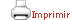Teaching plan for the course unit

(Short version)

 Català English CloseGeneral information

Course unit name: Bayesian Methods

Course unit code: 361222

Coordinator: XAVIER PUIG ORIOL

Department: Faculty of Economics and Business

Credits: 6

Single program: S

 Estimated learning time Total number of hours 150

 Face-to-face and/or online activities 60
 -  Lecture with practical component Face-to-face and online 30 -  IT-based class Face-to-face and online 30
 Supervised project 40
 Independent learning 50

 Learning objectives
 Referring to knowledge The main goal of this subject is to ensure students acquire a good understanding and mastery of Bayesian modelling in terms of both their theoretical and practical knowledge. This knowledge show ensure that students, when faced by a given objective or question, can, on the one hand, intervene in the design of the experiment or experiments needed to obtain the data that are the object of study and, on the other, analyse these data satisfactorily and draw conclusions to meet the objective or answer the question. And the specific objectives are: — Know the role of the a priori distribution, the role of the a priori reference distributions, and the transition from the a priori distribution to the a posteriori distribution. — Solve Bayesian inference problems analytically when using exponential family models and a priori conjugate distributions. — Use Monte Carlo methods, with specific software, to simulate the a posteriori distribution and know how to make inferences using these simulations. — Know the difference between hierarchical and non-hierarchical Bayesian models. — Know how to validate and compare Bayesian models and how to make Bayesian predictions.

 Teaching blocks

1. Bayesian model

1.1. Statistical model

1.2. The four problems of statistics

1.3. Likelihood

1.4. Bayesian model

1.5. A posteriori distribution

1.6. A priori and a posteriori predictive distribution

1.7. Choice of a priori distribution

2. Bayesian inference

2.1. A posteriori distribution as an estimator

2.2. Point estimation

2.3. Interval estimation

2.4. Tests of two hypotheses

2.5. Generalization of hypothesis testing

3. Bayesian computing

3.1. Need to integrate

3.2. Monte Carlo simulation based on Markov chains (MCMC)

3.3. Convergence of chains

4. Hierarchical models

5. Model validation and construction

Book

BOLSTAD, William M. Introduction to Bayesian Statistics. 2nd ed. Hoboken, N.J.: John Wiley, 2007

GELMAN, Andrew. Bayesian data analysis. 3rd ed. London: Chapman & Hall, 2014

CONGDON, Peter. Applied Bayesian Modelling. Wiley, 2014

KRUSCHKE, John K. Doing Bayesian Data Analysis: A Tutorial with R, JAGS, and Stan. Amsterdam: Academic Press, 2015.